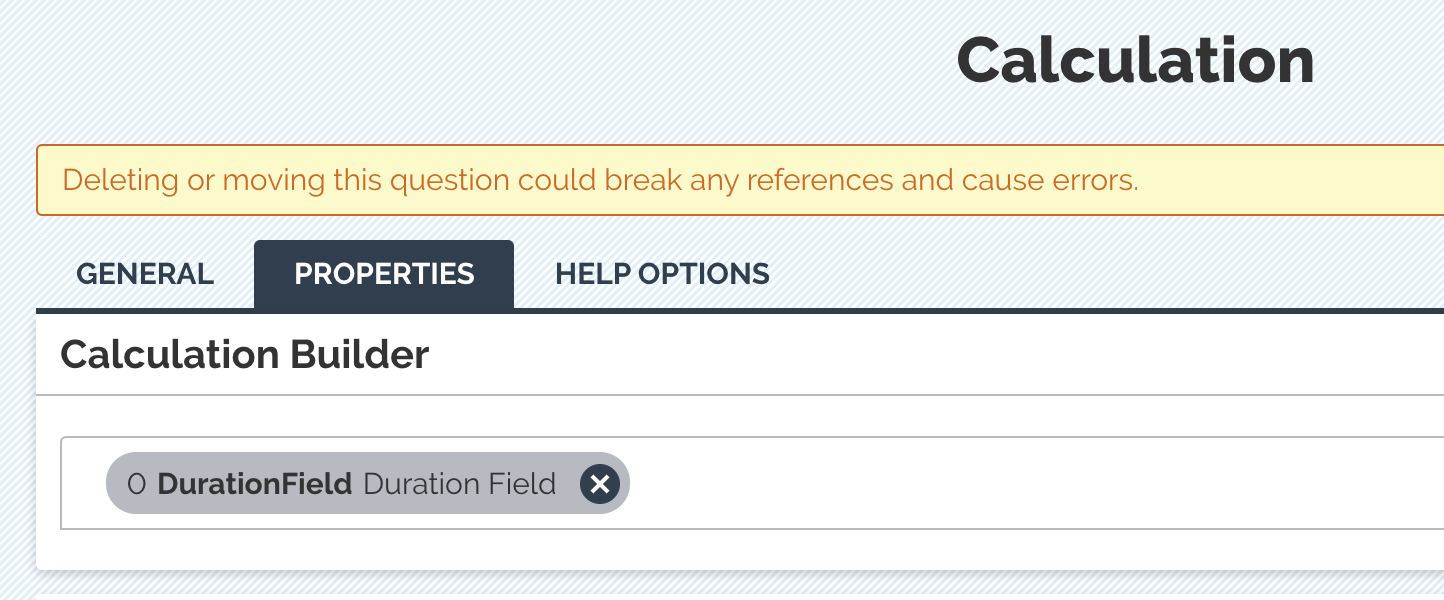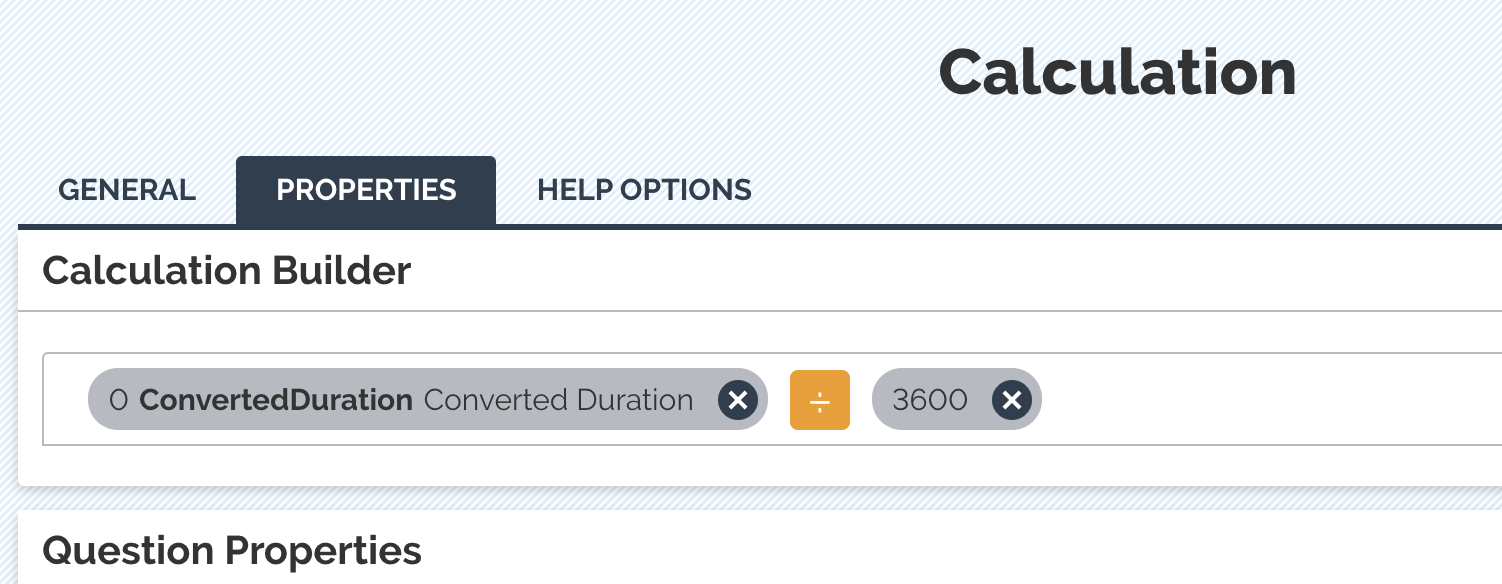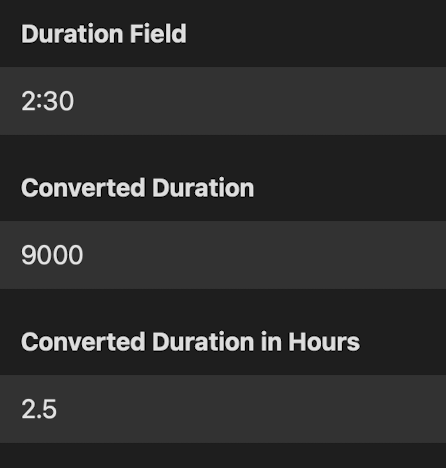# Best Practices

View Only

## Converting a Duration question to be a decimal number#### Ian Chamberlain08-26-2021 12:37• #### 1.  Converting a Duration question to be a decimal number

Posted 08-26-2021 12:37

Duration fields can be used to capture how long was spent doing a task, you can also calculate the duration between 2 date/time questions. There is sometimes a need to change the format of a duration question from it's default (hh:mm) to a decimal format.

For example, the duration field has 1:30 for 1 and a half hours but we need it to be shown as 1.5 instead. We can do this (and also convert to minutes or days if that is the requirement)

To get started, in the form builder we will need 1 duration field and 2 calculation questions set to "decimal".

The duration question can be left as default, no changes needed here.

The first calculation question will have ONLY the duration question as a part of the calculation, this will actually change your duration question into seconds. And from here, we can change it to anything that we want. NOTE: you can not do the division to calculate hours in this same question, we need to create a new calculation question to do that.The second calculation question will be the first calculation divided by how ever many seconds you want. If you are converting into hours, you will divide by 3600. If you want minutes, divide by 60.

Your second calculation question would look like this if you wanted hours.Now if you Deploy the form to test it out in the app, you will see this. Your Duration field, the first calculation question containing the number of seconds and the 3rd question converting it into decimal format. Now you can hide any of the questions that you don't need to see in the app using the "Hidden on Device" restriction.------------------------------
Ian Chamberlain
Implementation Specialist
ProntoForms
------------------------------

• #### 2.  RE: Converting a Duration question to be a decimal number

Posted 08-30-2021 13:44
I wish I had known this a while ago.  I'll have to redo one of my forms to include this conversion.

------------------------------
Scott Gilleland
Senior Field Service Engineer
Messer Cutting Systems
------------------------------